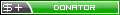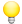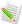Orbiter-ForumWhat optimal burn attitude profiles should look like
 User Name Remember Me? Password
 Register Blogs Orbinauts List Social Groups FAQ Projects Mark Forums Read

#1
 mjessickDonatorWhat optimal burn attitude profiles should look like
by mjessick 02-16-2009, 05:39 PM

For the problem of guiding a burn on orbit where the solution may not lie very close to being along the current instantaneous velocity vector (Orbiter autopilot's Prograde), you want to find some function of time for your burn attitude that ends at your desired final orbit and minimizes the burn time (and thus the fuel use).

Should I burn at constant attitude, or some constant attitude relative to the instantaneous velocity vector? What does an optimum attitude profile even look like?

Short answer: Almost anything you do on orbit is likely going to be "close enough."

Long answer: Depending on the assumptions you make, you can mathematically calculate the optimum attitude profile as a function of time during the burn. Assumptions that simplify the problem result in simpler answers (but to a problem that isn't quite the real one). Happily, these simpler solutions turn out to be very usable in practice though.

Here is a spectrum of sets of assumptions and solutions that get more complex as they go:

1. Hold the burn direction constant (assuming your burn is instantaneous even though it isn't). Solve the problem like an instantaneous delta-V and use that same attitude. Since your burn isn't really instantaneous, start it roughly half the burn duration early.

The Inertial Upper Stage (IUS) guidance used essentially this strategy, so you can do a lot with it. In practice, the solution is re-solved periodically during the burn using the delta-V remaining ("closed loop"), and this results in some attitude change during the burn.

2. "Linear Tangent Law": Make the tangent of the pointing angle above the horizon a linear function of time. (Optimal for no gravity, no atmosphere, in-plane trajectory.)

If you assume that tan(angle) = linear function of time is approximately eqivalent to angle= linear function of time I believe this is essentially the "Iterative Guidance Mode" (IGM) of the Saturn V, if you add in some careful coordinate transformations to make it work better. The optimality of an IGM nominal trajectory was quoted as around 5 to 25 kg of Saturn V payload relative to optimum. So these simplifying assumptions are likely to be good enough for most uses.

3. "Bi-Linear Tangent Law": The tangent of the pointing angle above the horizon is the ratio of two linear equations in time. (Optimal for constant gravity, no atmosphere, in-plane trajectory) Note: This is the 2 dimensional form of method 4 below.

(Also, if you don't care about how far you fly (the "range angle") for constant gravity and only constrain the final altitude and velocity vector, then you drop back to the linear tangent law (2 above). )

4. "Linear steering": burn with a time profile u(t) = A * t + B where A,B,u are three component cartesian vectors, with u the pointing direction vector. With this assumption of linear steering it turns out that you can integrate the burn trajectory analytically assuming a constant gravity vector (use an averaged gravity effect) and either constant thrust or constant thrust acceleration.

Ph.D. thesis: "Closed-Loop Nominal and Abort Atmospheric Ascent Guidance for Rocket-Powered Launch Vehicles," Greg Dukeman, (at MSFC)
http://smartech.gatech.edu/handle/1853/6820
See chapter 3 for the vacumn guidance
(Beware typo in eq. 3-3, I believe you should delete redundant first "T" in delta R sub T: that is, use f11 * A. Anyone who would like to try this can PM me for a starting implementation in C/C++.)

5. Or use one of the family of optimal solutions for assumptions of two body, inverse square gravity model, spherical planet, prescribed thrust acceleration profile (doesn't have to be constant, just known ahead of time), no atmosphere:

u_dd = - mu u / r^3 + (3 mu (r^T dot u) r ) /r^5

This is a differential equation for the vector u (the optimal pointing direction) where u_dd is u "double dot", the second derivative of u. r^T is the transpose of the radius vector of magnitude r, mu the gravitational parameter. This is the solution family found via the Calculus of Variations for the more accurate modelling assumptions mentioned.

All you have to dois pick u(0), u_d(0) (the starting u and first derivative of u), and burn duration, then integrate this equation to follow the optimum (minimum time) burn direction (u(t)) over the burn to the final orbit. Of course, the trick is finding the initial conditions that go to the final orbit that is the one that you wanted.That is, following this type of profile assures you are following an optimal path to some orbit within the assumptions above, but probably not the one you wanted to get to ;0

This is the second Brown and Johnson paper:
http://www.research.ibm.com/journal/...ibmrd1104D.pdf

I find the earlier "Real-Time Optimal Guidance" by Brown and Johnson more readable, but haven't found it available online without fee yet.

From "Real-Time Optimal Guidance", the improvement from going to this level of sophistication vs. IGM was slight for a Saturn V ascent, although shows more improvement in some disturbance cases.
_________

Summary: when you try to go beyond making some severe simplifying assumptions, it gets much more complicated to describe the optimum profile for the pointing direction during a burn. And you get less and less benefit from the increased complication.

So just use profiles from 1, 2, or 4 above, although 5 is really slick.

Note: These are all optimal for various assumptions that include "no atmosphere." Lift and drag complicate the mathematics immensely.
Views 4958 Comments 1
 Thanked by:03-07-2009, 11:25 PM #2 tfr001 OrbinautSaturn 5 IGM Actually, the Saturn 5 IGM was something like your #4, and only used on second and third stage burns. First stage *was* one of those simple tip-it-over-at-some-linear-rate things, designed to minimize aerodynamic forces. See: http://ntrs.nasa.gov/archive/nasa/ca...1964003582.pdf (I'm currently modeling Saturn 5 in Excel, so I've been doing a lot of research...)Thanked by:

 Article ToolsShow Printable VersionEmail this PagePosting Rules BB code is On Smilies are On [IMG] code is On HTML code is Off You may not post new threads You may not post replies You may not post attachments You may not edit your posts
 Forum Jump User Control Panel Private Messages Subscriptions Who's Online Search Forums Forums Home Orbiter-Forum.com     Announcements     Meets & Greets Orbiter Space Flight Simulator     Orbiter Web Forum         OFMM         Orbiter Forum Space Station         Simpit Forum     General Questions & Help     MFD Questions & Help     Hardware & Software Help     Tutorials & Challenges     Orbiter SDK     Orbiter Visualization Project     Orbiter Beta » Orbiter Project Orbiter Addons     OrbitHangar Addons & Comments     Addons     Addon Development     Addon Requests     Addon Support & Bugs         Addon Developer Forums             Project Apollo - NASSP     Orbiter Lua Scripting Far Side of the Moon     Spaceflight News     Math & Physics     Astronomy & the Night Sky     Backyard Rocketry     Brighton Lounge     International Forum

All times are GMT. The time now is 06:45 PM.

About Us | Rules & Guidelines | TOS Policy | Privacy Policy

Orbiter-Forum is hosted at Orbithangar.com